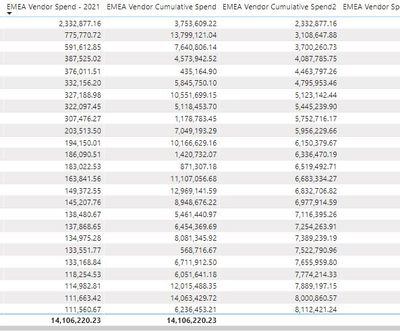cancel
Showing results for
Did you mean:

Fabric is Generally Available. Browse Fabric Presentations. Work towards your Fabric certification with the Cloud Skills Challenge.Anonymous
Not applicable

## Quick Measure - Running Total Sorting issue

I am trying to create a Pareto Chart which shows the running Total of Spend in percentge as Line.

The Columns are sorted by Total Spend for each category (MM, PPP, SS etc) in Desc orderI used Quick Measure option on Sum("Spend") field to create a "Running Total". When this is added as a Line Value, the data points are appearing sorted by the Category ( notice VVV has 100% and its the 4 bar, ).

The Cumulative of Spend (Running Total) should happened by the Total Spend sorted in Desc order, not by Category Text!
Here is the DAX created by Quick Measure:

```Sumof Line Amount running total in Category

= CALCULATE(

SUM('Spend'[Line Amount]),

FILTER(
ALLSELECTED('Spend'[Category]),

ISONORAFTER('Spend'[Category],
MAX('Spend'[Category]),
DESC)
)
)```

1 ACCEPTED SOLUTIONCommunity Champion

@Anonymous

Hi, Try with this Code: (Assuming that the Graph was sorted by Spend)

```Spend running total =
VAR spend =
CALCULATE ( SUM ( Table2[Spend] ) )
RETURN
CALCULATE (
SUM ( Table2[Spend] ),
FILTER (
ALL ( Table2[Category] ),
CALCULATE ( SUM ( Table2[Spend] ) >= spend )
)
)```Lima - Peru
8 REPLIES 8Microsoft

Hi @Anonymous

Does this measure get close to what you need?

```Sumof Line Amount running total in Category =
CALCULATE(
SUM('Spend'[Line Amount]),
FILTER(
ALL('Spend'),
'Spend'[Category]<=MAX('Spend'[Category])
)
)```

Proud to be a Datanaut!Anonymous
Not applicable

Thank you for the resposes!

1) Sorting on the line will not working, because its a Pareto Chart, that should Columns sorted by Top Spend. The Line should have Running total for each Bar.

2) Phil, I tried your Formula, its giving similar output. Here is the output:Ideally, the output shoudl look like this:Community Champion

@Anonymous

Hi, Try with this Code: (Assuming that the Graph was sorted by Spend)

```Spend running total =
VAR spend =
CALCULATE ( SUM ( Table2[Spend] ) )
RETURN
CALCULATE (
SUM ( Table2[Spend] ),
FILTER (
ALL ( Table2[Category] ),
CALCULATE ( SUM ( Table2[Spend] ) >= spend )
)
)```Lima - PeruNew Member

Hi, if the spend for some of the categories is exactly the same (the column spend has duplicate values), then this formula is not useful as it shows the same Running Total for the duplicate values. Do you have any suggestion how to treat this issue? Thanks.Super User

Hi,

Yes, there is a way to solve for that.  Share some data and also the expected result.

Regards,
Ashish Mathur
http://www.ashishmathur.comHelper IV

I just found this thread and it solved my problem! However, would you know why I am not getting a total? The initial Cumulative Spend column was working but it was based on an alphabetical sort, where I wanted it based on descending spend sort, which your post did. However my first one gave me a total and the new one is not. Any ideas?Anonymous
Not applicable

Yes! This worked for me. After two days of googling, I finally found this solution.

Thank you!Microsoft

@Anonymous

You can change the sort order as below. Not sure if it is your expected result.Best Regards,

HerbertAnnouncements#### Power BI Monthly Update - November 2023

Check out the November 2023 Power BI update to learn about new features.#### Fabric Community News unified experience

Read the latest Fabric Community announcements, including updates on Power BI, Synapse, Data Factory and Data Activator.#### The largest Power BI and Fabric virtual conference

130+ sessions, 130+ speakers, Product managers, MVPs, and experts. All about Power BI and Fabric. Attend online or watch the recordings.Top Solution Authors
Top Kudoed Authors
Users online (2,107)